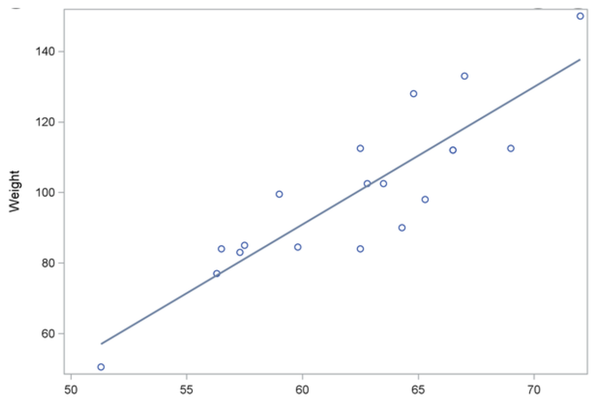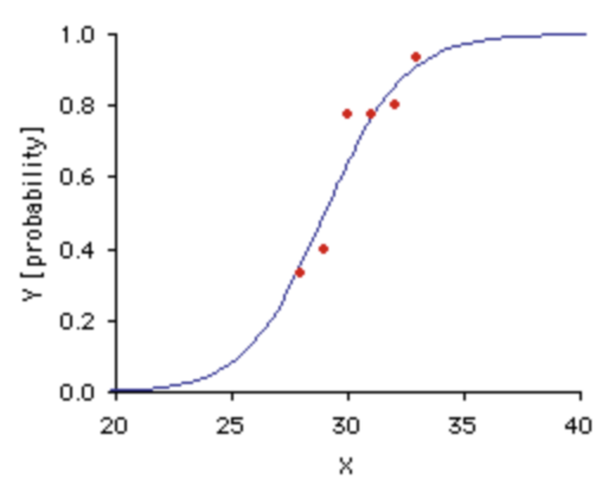# What is logistic regression?

Logistic regression is used to predict the binary outcome from the given set of continuous predictor variables.

Logistic regression is used to obtain odds ratio in the presence of more than one explanatory variable. The procedure is quite similar to multiple linear regression, with the exception that the response variable is binomial. The result is the impact of each variable on the odds ratio of the observed event of interest. Logistic regression, also known as logit regression or logit model, is a mathematical model used in statistics to estimate (guess) the probability of an event occurring having been given some previous data. Logistic regression works with binary data, where either the event happens (1) or the event does not happen (0).

Logistic regression is a supervised classification algorithm. It is a discriminative algorithm, meaning it tries to find boundaries between two classes. It models the probabilities of one class.

In linear regression (y=mx + c) our output(y) can be from -inf to +inf , but in logistic we want our output to be probabilities ( between 0 to 1 ).

Here comes the logistic function(sigmoid function), y = 1/(1+e^(-x))

Output of linear regression:Output of logistic regression(sigmoid curve):The equation for simple logistic regression is:

y = e^(mx+c)/(1 + e^(mx+c))

The loss function used in logistic regression is log loss

log_loss = $\sum_{i=0}^{n} (-y_ilog(y_i’)) - (1-y_i)(log(1-y_i’)))$

here, n is the number of training instances, y is the actual value and y’ is the predicted value.